﻿ 考虑了交箱时间不确定性的场桥堆存作业优化文章快速检索 高级检索

Stockpiling operating optimization for yard crane with containers delivery time uncertainty
SHAO Qian-qian, , , JIN Zhi-hong
College of Transportation Management, Dalian Maritime University, Dalian 116026, China
Abstract:The uncertainty of customer delivery time has a direct effect on stockpiling efficiency and status of exported containers, which may lead to increasing the yard crane moving distance and the follow-up loading relocations. In this paper, Markov forecast is applied to obtain a generalized delivery sequence. Based on that, a two-stage model is formulated for storage and crane moving path optimization, considering the actual operations of container terminals. In addition, static algorithm for making the initial plan and dynamic algorithm for making the real-time optimization are developed respectively. Several sets of experimental results demonstrated the effectiveness and practicability of the model and the algorithm by the comparison with different stockpiling strategies. The model and algorithm proposed in this paper provide decision support for real-time scheduling scheme of crane yard.
Key words: container     yard crane scheduling     relocation     generalized delivery sequence     Markov chain

0 引言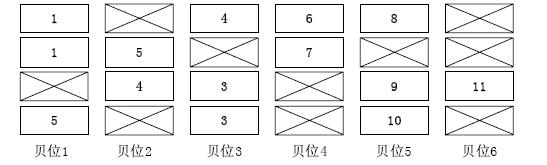图 1 街区示意图

1)已知待分配的街区;

2)所有待堆存集装箱箱型相同,如均为20英尺标准集装箱;

3)客户的集装箱交箱时间、目的港、重量等信息已知; 同一客户的集装箱交箱时间、目的港、重量相同(否则视为不同客户);

4)基于装船作业实务,翻箱数量按照长途箱压短途箱、 重箱压轻箱的规则进行加权计算;

5)场桥的移动距离以贝位为单位计算,初始位置为贝位1;

6)根据每一个客户的集港堆存方案,场桥按照贝位顺次作业. 1.2 第一阶段模型 1.2.1 符号说明

$I$: 堆场堆垛栈的额定高度;

$N$: 待进港客户总数;

$M$: 一个充分大的正数; $U_n$: 客户$n$的待进港集装箱数量;

$F_n$: 客户$n$参与翻箱的集装箱数量,也即$F_{n}=U_{n}mbox{mod}\ I$;

$T$: 所有客户参与翻箱的集装箱构成的翻箱栈的总数, 也即$T=[\sum_{n=1}^{N}F_n-(\sum_{n=1}^{N}F_n)mbox{mod}\ I]/I+1$;

$O_n$: 客户$n$的进场顺序;

$d_n$: 客户$n$集装箱的目的港序号;

$w_n$: 客户$n$集装箱的重量等级,最大重量等级为$W$;

$c_n$: 客户$n$集装箱的堆存优先级,其中$c_n=Wd_n+w_n$;

$slot(t,i)$: 位于第$t$个翻箱栈第$i$层的箱位.

$s_{nti}$: 第$t$个翻箱栈第$i$层是否被第$n$个客户的集装箱占据,即:

$s_{nti}=\left\{ \begin{array}{ll} 1 ,& \mbox{当$slot(t,i)$被客户$n$的集装箱占据;}\\ 0 ,& \mbox{其他. } \end{array} \right.$

$n=1,2,\cdots,N;t=1,2,\cdots,T;i=1,2,\cdots,I.$

$R_{t(i-z)}(s_{nti},s_{nt(i-z)})$: 堆放在$slot(t,i)$与$slot(t,i-z)$ 上的集装箱是否有翻箱,如果前者的优先级小于后者,则产生翻箱,即:

$R_{t(i-z)}(s_{nti},s_{nt(i-z)})=\left\{ \begin{array}{ll} 1,&\mbox{当分配到$slot(t,i)$的集装箱权值$c_n$小于分配到$slot(t,i-z)$上的集装箱;}\\ 0 ,&\mbox{其他. } \end{array} \right.$

$t=1,2,\cdots,T;i=2,3,\cdots,I;z=1,2,\cdots,i-1.$ 1.2.2 目标函数与约束条件

 $$P_1=\min\sum_{t=1}^T\sum_{i=2}^I\sum_{z=1}^{i-1} R_{t(i-z)}(s_{nti},s_{nt(i-z)})$$ (1)

 $$\sum_{t=1}^T\sum_{i=1}^I s_{nti}=F_n ,\qquad n=1,2,\cdots,N$$ (2)
 $$\sum_{n=1}^N s_{nti}\le1 ,\qquad t=1,2,\cdots,T;i=1,2,\cdots,I$$ (3)
 $$\sum_{n=1}^N s_{nti} \le \sum_{n=1}^N s_{nt(i-1)},\qquad t=1,2,\cdots,T;i=2,3,\cdots,I$$ (4)
 $$\sum_{n=1}^N O_n s_{nti} +\bigg(1-\sum_{n=1}^N s_{nti}\bigg)M >\sum_{n=1}^N O_n s_{nt(i-1)}, \qquad t=1,2,\cdots,T;i=2,3,\cdots,I$$ (5)

$$R_{t(i-z)}(s_{nti},s_{nt(i-z)})=\bigg\lceil \frac{V}{\exp(V)} \bigg\rceil$$

 $$V=\sum_{n=1}^N S_{nti} \bigg\lceil \exp\bigg(\sum_{n=1}^N c_n s_{nt(i-z)} - \sum_{n=1}^N c_n S_{nti} \bigg)-1\bigg\rceil$$ $$t=1,2,\cdots,T;i=2,3,\cdots,I;z=1,2,\cdots,i-1$$ (6)

$K$: 街区内的贝位总数;

$J$: 每一贝堆垛的栈数;

$ZE_n$: 每个客户不参与翻箱的"整栈"数, 也即$E_n=(U_n-F_n)/I$;

$h_{tjk}$: 第$k$贝第$j$栈是否被$H_t$占据, 即:

$h_{tjk}=\left\{ \begin{array}{ll} 1 ,&\mbox{当$k$贝中第$j$栈被$H_t$占据;}\\ 0 ,&\mbox{其他. } \end{array} \right.$\\ $t=1,2,\cdots,T;j=1,2,\cdots,J;k=1,2,\cdots,K.$\\ $g_{njk}$:

$g_{njk}=\left\{ \begin{array}{ll} 1 ,&\mbox{当$k$贝中第$j$栈被$Z_n$占据;}\\ 0,&\mbox{其他. } \end{array} \right.$\\ $n=1,2,\cdots,N;j=1,2,\cdots,J;k=1,2,\cdots,K.$\\ $e_{nk}$:

$e_{nk}=\left\{ \begin{array}{ll} 1,&\mbox{当第$k$贝存在客户$n$的集装箱,即$\sum_{j=1}^J g_{njk} +\sum_{t=1}^T \sum_{j=1}^J (h_{tjk} \sum_{i=1}^I s_{nti}) \geq 0$;}\\ 0 ,&\mbox{其他. } \end{array} \right.$

$n=1,2,\cdots,N;k=1,2,\cdots,K.$

$E_{nkp}$: 客户$n$在堆存时第$k$贝与第$p$贝是否存在顺次移动距离, 即:

$E_{nkp}\!=\!\left\{ \begin{array}{ll} 1,&\mbox{当客户$n$在堆存时第$k$贝与第$p$贝是否存在顺次移动距离,即$e_{nk}e_{np}(1\!\!+\!\! \sum_{q=k+1}^{p-1}e_{nq})\!=\!1$;}\\ 0,&\mbox{其他. } \end{array} \right.$

$n=1,2,\cdots,N;k=1,2,\cdots,K-1;p=k+1,K+2,\cdots,K.$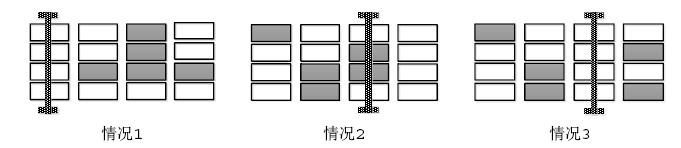图 2 三种切换作业

$O_n^{\prime}$: 第$n$个进场的客户编号,$O_{O_n}^{\prime}=n$, $n=1,2,\cdots,N.$

${\rm Max}_n$: 第$n$个进场的客户堆存的贝位序号最大值, 其值为$\max\{ke_{(O_n^{\prime})k}\}$,$n=1,2,\cdots,N$.

${\rm Min}_n$: 第$n$个进场的客户堆存的贝位序号最小值, 其值为$\min\{ke_{(O_n^{\prime})k}\}$,$n=1,2,\cdots,N$.

${SW}_n$:第$n$个进场客户的场桥作业结束所在贝位序号, 为一个递推序列, 其中:

${SW}_1={\rm Max}_1$,

${SW}_n=\left\{ \begin{array}{llll} {\rm Min}_n ,&\mbox{当${SW}_{n-1}\geq {\rm Max}_n$;}\\ {\rm Max}_n,&\mbox{当${SW}_{n-1}\leq {\rm Min}_n$;}\\ {\rm Min}_n,&\mbox{当${SW}_{n-1}-{\rm Min}_n\geq {\rm Max}_n-{SW}_{n-1}$;}\\ {\rm Max}_n ,&\mbox{当${SW}_{n-1}-{\rm Min}_n\leq {\rm Max}_n-{SW}_{n-1}$;}\\ \end{array} \right.$

$n=2,3,\cdots,N.$

${DS}_n$:第$n-1$个进场客户与第$n$个进场客户的场桥切换作业移动距离, 为一个递推序列, 其中:\\ ${DS}_1={\rm Min}_1$,

$DS_n=\left\{ \begin{array}{llll} {SW}_{n-1}-{\rm Max}_n,&\mbox{当${SW}_{n-1}\geq {\rm Max}_n$;}\\ {\rm Min}_n-{SW}_{n-1},&\mbox{当${SW}_{n-1}\leq {\rm Min}_n$;}\\ \min\{{SW}_{n-1}-{\rm Min}_n,{\rm Max}_n-{SW}_{n-1}\},&\mbox{当${\rm Min}_n<{SW}_{n-1}<{\rm Max}_n$;}\\ \end{array} \right.$

$n=2,3,\cdots,N.$ 1.3.2 目标函数与约束条件

 $$P_2=\min\bigg(\sum_{n=1}^N {DS}_n+\sum_{n=1}^N \sum_{k=1}^K \sum_{p=k+1}^K (p-k)E_{nkp}\bigg)$$ (7)
 $$\sum_{j=1}^J \sum_{n=1}^N g_{njk} + \sum_{j=1}^J \sum_{t=1}^T h_{tjk}\leq J,\qquad k=1,2,\cdots,K$$ (8)

 $$\sum_{k=1}^K \sum_{j=1}^J g_{njk}=ZE_n,\qquad n=1,2,\cdots,N$$ (9)
 $$\sum_{k=1}^K \sum_{j=1}^J h_{tjk}=1,\qquad t=1,2,\cdots,T$$ (10)
 $$\sum_{n=1}^N g_{njk} \sum_{t=1}^T h_{ijk}\leq1,\qquad j=1,2,\cdots,J;k=1,2,\cdots,K$$ (11)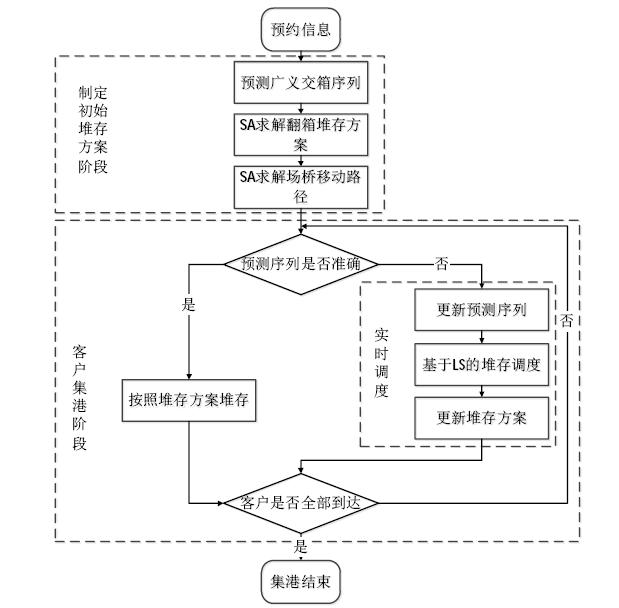图 3 算法流程图
2.1 初始堆存方案算法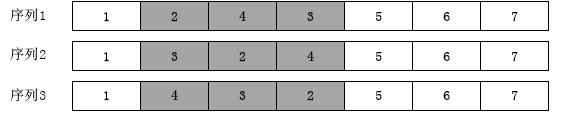图 4 客户到达序列示意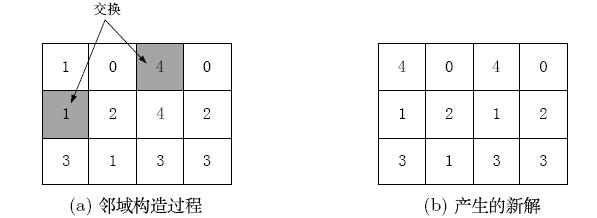图 5 第一阶段模型解的邻域构造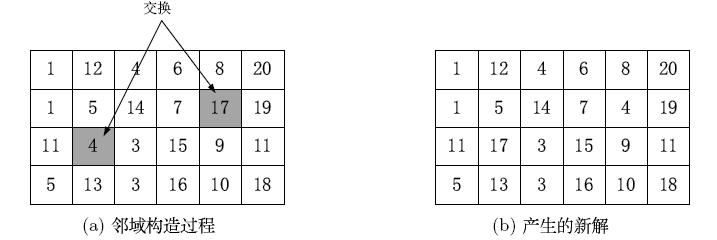图 6 第二阶段模型解的邻域构造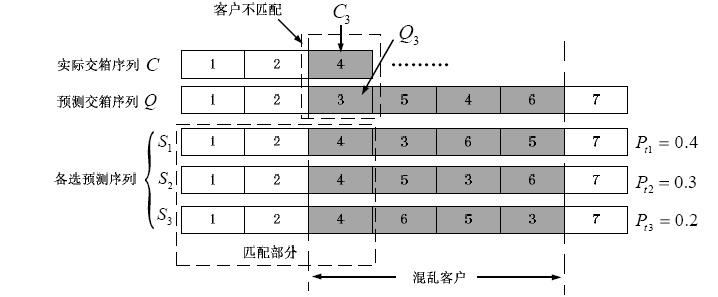图 7 预测交箱序列的更新

Step 1 按照$Q^{\prime}$调整$Pl_n$内每一栈集装箱的堆垛顺序,设定正整数$\beta$,$\alpha$;

Step 2 若$\alpha=\beta$,算法结束; 否则基于方案$Pl_n$在集合$H$ 内随机选取两个不同的"翻箱栈"$H_{t1}$和$H_{t2}$,并分别在$H_{t1}$和$H_{t2}$内随机选取两个编号不同的未集港客户的集装箱进行交换形成$H_{t1}^{\prime}$和$H_{t2}^{\prime}$,并根据$Q^{\prime}$调整该两栈内的堆存顺序;

Step 3 计算翻箱量差值$\Delta f=f(H_{t1}^{\prime})+f(H_{t2}^{\prime})-f(H_{t1})-f(H_{t2})$, 若$\Delta f\leq0$,则接受新解,并令$\alpha=0$,否则保留原解,并令$\alpha=\alpha+1$,执行Step 2;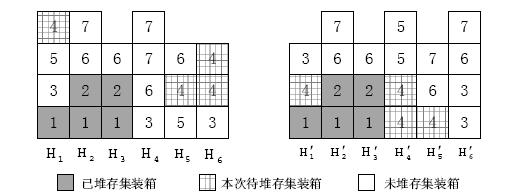图 8 "翻箱栈"调度前后堆存情况对比图

Step 1 设定正整数$\lambda$,$\alpha=0$;

Step 2 若$\alpha=\lambda$,算法结束; 否则在方案$Pl_n^{\prime}$ 中随机选取两个贝位$K_1$和$K_2$,并分别在$K_1$和$K_2$ 内随机选取两个尚未堆存集装箱且编号不同的栈进行交换,产生新堆存方案$Pl_n^{\prime\prime}$;

Step 3 基于$Q^{\prime}$计算方案$Pl_n^{\prime\prime}$与方案$Pl_n^{\prime}$的大车移动 距离差值$\Delta g=f(Pl_n^{\prime\prime})-f(Pl_n^{\prime})$,若$\Delta g\leq0$,则接受新解,并令$\alpha=0$, 否则保留原解新解,并令$\alpha=\alpha+1$,执行Step 2;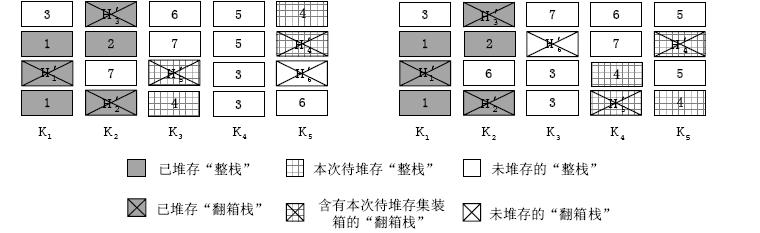图 9 整体堆存情况调度前后对比图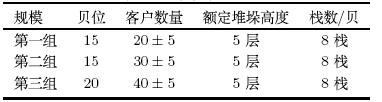图 10 算法收敛图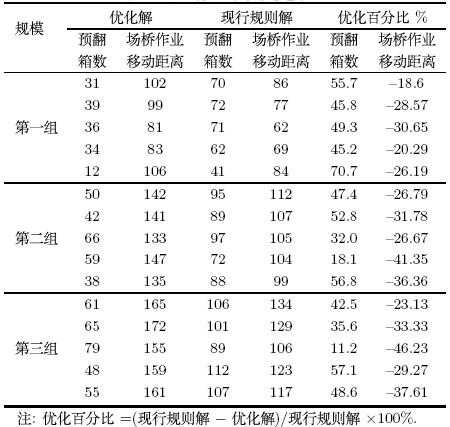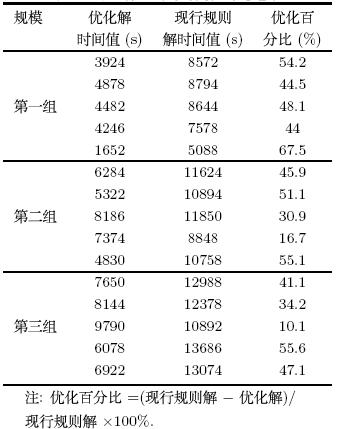3.2 实时调度优化策略对比 3.2.1 实时调度优化策略 策略1: 不变策略

Step 1 若$r=F_n$,调度结束,否则令$r=r+1$,执行Step 2;

Step 2 依次判断$H_t$是否有空箱位,若存在则计算$\Delta l=l(B_{C_nr},H_{tn})$,并将集装 箱$B_{C_nr}$放入$\Delta l$最小值所在的栈,若该栈不唯一,则等概率选取其中一个,执行Step 1;

Step 1 若$r={ZE}_n$,调度结束,否则令$r=r+1$,执行Step 2;

Step 2 依次判断${BA}_k$是否有空栈位, 若存在空栈位则计算$d(Z_{C_nr},BA_k)$,将$Z_{C_n1}$放入 函数值最小的贝位$BA_k^{\prime}$ 中,若该贝位不唯一, 则等概率选取其中一个,执行Step 1. 3.2.2 实验设计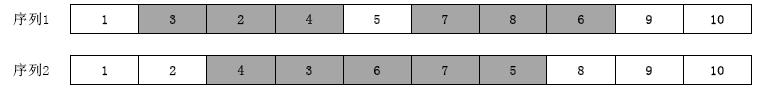图 11 混乱序列
3.2.3 结果分析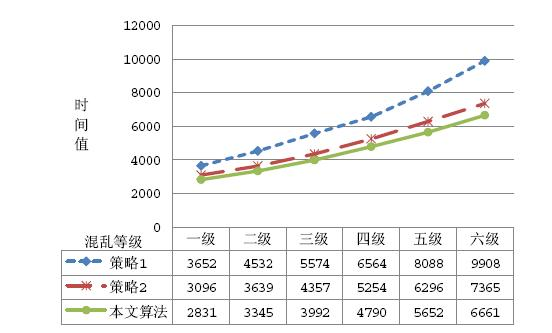图 12 不同混乱等级下与两种调度策略的对比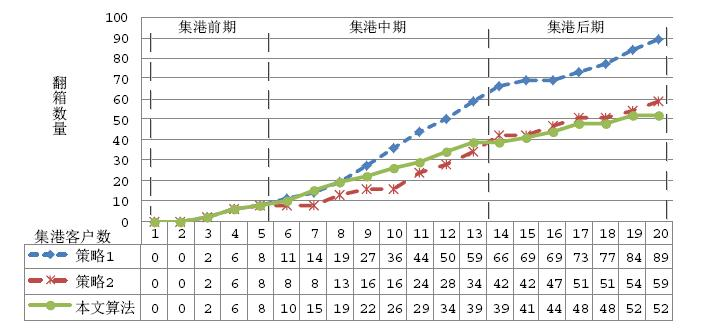图 13 集港过程中与两种调度策略的预翻箱数对比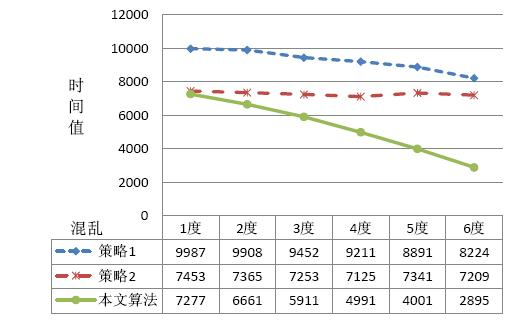图 14 不同混乱度下与两种调度策略的对比

  Kim K Y, Kim K H. A routing algorithm for a single transfer crane to load export containers onto a containership[J]. Computers & Industrial Engineering, 1997, 33(3-4): 673-676.  Kim K H, Kim K Y. An optimal routing algorithm for a transfer crane in port container terminals[J]. Transportation Science, 1999, 33(1): 17-33.  Kim K Y, Kim K H. Heuristic algorithm for routing yard-side equipment for minimizing loading times in container terminals[J]. Naval Research Logistics, 2003, 50(5): 498-514.  Narasimhan A, Palekar U S. Analysis and algorithms for the transtainer routing problem in container port operations[J]. Transportation Science, 2002, 36(1): 63-78.  Kim K H, Park Y M. A crane scheduling method for port container terminals[J]. European Journal of Operational Research, Part E, 2004, 156: 752-768.  Ng W C, Mark K L. An effective heuristic for scheduling a yard crane to handle jobs with different ready times[J]. Engineering Optimization, 2005, 37(8): 867-877.  韩晓龙. 集装箱港口龙门吊的最优路径问题[J]. 上海海事大学学报, 2005, 26(2): 39-41. Han Xiaolong. Routing problem of transfer crane at container terminals[J]. Journal of Shanghai Maritime University, 2005, 26(2): 39-41.  Kim K H, Lee J S.Satisfying constraints for locating export containers in port container terminals[C]// International Conference on Computational Science and Its Applications, 2006: 564-573.  何军良, 宓为建, 严伟.基于爬山算法的集装箱堆场场桥调度[J].上海海事大学学报, 2007, 28(4): 11-15. He Junliang, Mi Weijian, Yan Wei. Container yard crane scheduling based on hill-climbing algorithm[J]. Journal of Shanghai Maritime University, 2007, 28(4): 11-15.  周鹏飞, 方波. 基于随机交箱序列的集装箱堆场出口箱箱位优选[J]. 沈阳工业大学学报, 2011, 33(6): 678-685. Zhou Pengfei, Fang Bo. Slot allocation optimization for export container in container storage yard based on stochastic delivery sequence[J]. Journal of Shenyang University of Technology, 2011, 33(6): 678-685.  周鹏飞, 李丕安. 不确定条件下集装箱堆场出口箱具体箱位优选[J]. 工业工程, 2013, 16(1): 25-30. Zhou Pengfei, Li Pian. Slot allocation for export containers in container yard with arrival and leaving time uncertainties delivery sequence[J]. Industrial Engineering Journal, 2013, 16(1): 25-30.
0

#### 文章信息

SHAO Qian-qian, XU Qi, BIAN Zhan, JIN Zhi-hong

Stockpiling operating optimization for yard crane with containers delivery time uncertainty

Systems Engineering - Theory & practice, 2015, 35(2): 394-405.